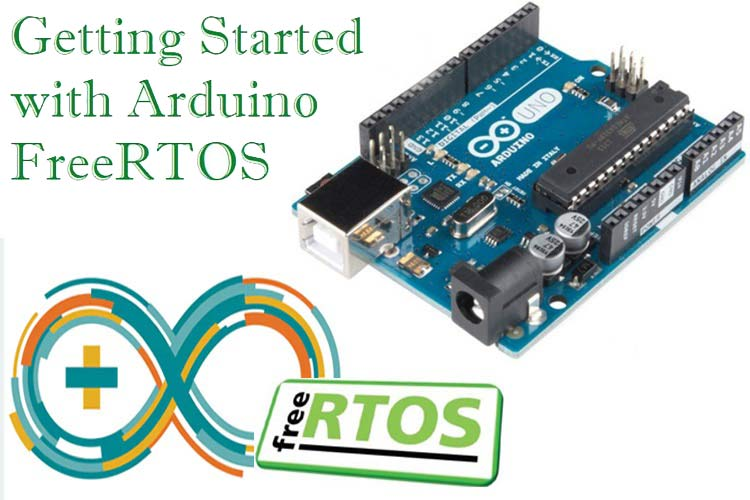## How to convert binary fractions to decimal ( fixed point arithmetics )

```Example for n = 110.101

Step 1: Conversion of 110 to decimal
=> 1102 = (1*22) + (1*21) + (0*20)
=> 1102 = 4 + 2 + 0
=> 1102 = 6
So equivalent decimal of binary integral is 6.

Step 2: Conversion of .101 to decimal
=> 0.1012 = (1*1/2) + (0*1/22) + (1*1/23)
=> 0.1012 = 1*0.5 + 0*0.25 + 1*0.125
=> 0.1012 = 0.625
So equivalent decimal of binary fractional is 0.625

Step 3: Add result of step 1 and 2.
=> 6 + 0.625 = 6.625```
```Example for n = 4.47 k = 3

Step 1: Conversion of 4 to binary
1. 4/2 : Remainder = 0 : Quotient = 2
2. 2/2 : Remainder = 0 : Quotient = 1
3. 1/2 : Remainder = 1 : Quotient = 0

So equivalent binary of integral part of decimal is 100.

Step 2: Conversion of .47 to binary
1. 0.47 * 2 = 0.94, Integral part: 0
2. 0.94 * 2 = 1.88, Integral part: 1
3. 0.88 * 2 = 1.76, Integral part: 1

So equivalent binary of fractional part of decimal is .011

Step 3: Combined the result of step 1 and 2.

Final answer can be written as:
100 + .011 = 100.011```

## RTOS on ArduinoProfessional software developers of time critical code often use a real time operating system (RTOS) in their work. The reason is that the OS is taking care of timing and scheduling tasks with higher priority. Now you can easily use an RTOS on your Arduino as well! Read Jain Risbah’s article over at circuitdigest: https://circuitdigest.com/microcontroller-projects/arduino-freertos-tutorial1-creating-freertos-task-to-blink-led-in-arduino-uno

## How to check for a NAN (Not A Number) in C++ in embedded systems without exception handlers

Sometimes there are bugs or special corner conditions that makes a NAN (Not A Number) occur in code compiled from a C++ source and executed on an embedded systems without memory to run exception handlers.

Here is what @Jalf writes over at StackExchange:

```According to the IEEE standard, NaN values have the odd property that comparisons involving them are always false. That is, for a float f, `f != f` will be true only if f is NaN.

Note that, as some comments below have pointed out, not all compilers respect this when optimizing code.

For any compiler which claims to use IEEE floating point, this trick should work.```

## If you inplement a function in a separate .cpp file , how do you access objects instantiated in main from functions implemented that file?

Sometimes there is a need to spread code into several .cpp files to avoid clutter (for example main.cpp, other1.cpp, other 2.cpp) . So if you implement a function in a separate .cpp file, how do you access objects instantiated in main?

Solution: use extern

```other1.cpp:

extern ObjectType objectname

void doSomething (void)
{
objectname.method();
}```